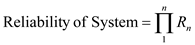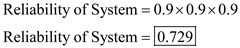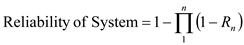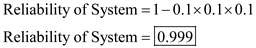# Quiz 10: Managing Engineering Design

Business

Systems engineering and new product development should be divided into multiple phases to emphasize the efforts being directed to complete the project into a particular area. The division of the phases is created so that there is logical flow between the phases. It also helps in efficient utilization of available resources and completion of project within time. Thus, division of project into stages provides a logical continuum of tasks that need to be completed with least possible iterations.

a) If the three components are arranged in series, calculate reliability of the entire system using following formula:Where, R n represents the reliability of the n th component in the system. In this case, the system has three components each with reliability of 0.9, therefore, total reliability of the systemThus, the system is 72.9% reliable. b) If three components are arranged in parallel, calculate reliability of the entire system using following formula:Where, R n represents reliability of n th component in the system. In this case, the system has three components each with reliability of 0.9, therefore, the total reliability of the system is calculated as follows:Thus, the system is 99.9% reliable. c) If two components are used in parallel and one component in series to the pair in parallel, calculate total reliability of the system in following manner:Thus, the system is 89.1% reliable. d) If two components are placed in series, and that pair is placed in parallel with another component, calculate total reliability of the system in following manner:Thus, the system is 98.1% reliable.

The design work done in the technical feasibility stage is not in detail of how the product will be manufactured or of the particular features of the product, instead focuses on determining whether the project can be completed within time and resources or not. However, the work done in new product development stage will delve more into the details and specific features of the product etc.